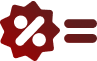# Simple Interest Calculator

## Simple interest is calculated by multiplying the rate of interest by the principal and duration

Home

Math

Interest Calculator

=

### Principal Amount: 100## Simple Interest Formula## Simple Interest Rate Calculator table

Principal : 100

Interest Rate : 10%

## Frequently Asked Questions on Interest Calculator